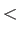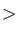### Keyword VELOCITIES

This keyword specifies the initial nuclear velocities and, therefore, the initial temperature of a BOMD simulation.
Options:
 RANDOM Random velocities from a uniform distribution. ZERO Initial velocities are set to zero. READ Initial velocities are read from the input file. P=0 Adjust the initial velocities to zero linear momentum. LP=0 Adjust the initial velocities to zero linear and angular momentum. T=RealSpecifies the temperature [K] to which the initial velocities are scaled. Default is 300 K.
``` VELOCITIES READ